## Example Questions

### Example Question #1 : How To Subtract Odd Numbers

Assumeandare both odd whole numbers and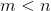.

What is a possible solution for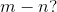Explanation:

An odd whole number minus an odd whole number will result in an even whole number. Since, the subtraction will result in a negative even whole number. The only answer that fits these requirements is.

### Example Question #91 : Integers

Choose the answer below which best solves the following equation: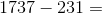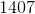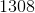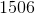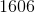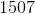Explanation: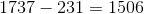If you have trouble with problems like this, try stacking the numbers, and subtracting one place at a time.  First, seven minus one is six, so that's your ones digit.  Next, three minus three is zero, so that's your tens digit.  Seven minus two is five, so that's your hundreds digit, and finally, one minus zero is one so that's you're thousands digit.

### Example Question #92 : Integers

Solve the following: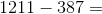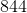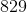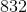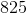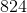Explanation:

To solve, simply subtract.  If you have trouble subtracting, try splitting the numbers up and adding your results: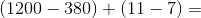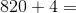### Example Question #93 : Integers

Solve the following: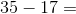Explanation:

To perform this operation, simply subtract.  If you are having trouble, you can split the problem into two easier to solve portions, by splitting the numbers up: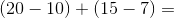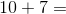### Example Question #94 : Integers

Solve the following: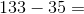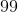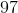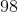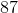Explanation:

To solve, simply subtract.  If you have trouble subtracting, you can split the problem up and add your results back together: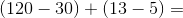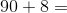### Example Question #95 : Integers

Solve the following: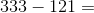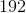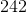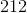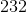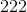Explanation:

To solve, simply subtract.  If you have trouble subtracting, you can split the numbers up, subtract, and add your results: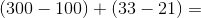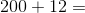Tired of practice problems?

Try live online GRE prep today.